# Do we do the vegetation data clustering analysis right?

Vegetation data analysis is one of the crucial issues we deal with in almost all researches we do in vegetation Science. One of the most common vegetation data analyses is the clustering analysis. In this article, I am going to focus on the clustering tendency issue.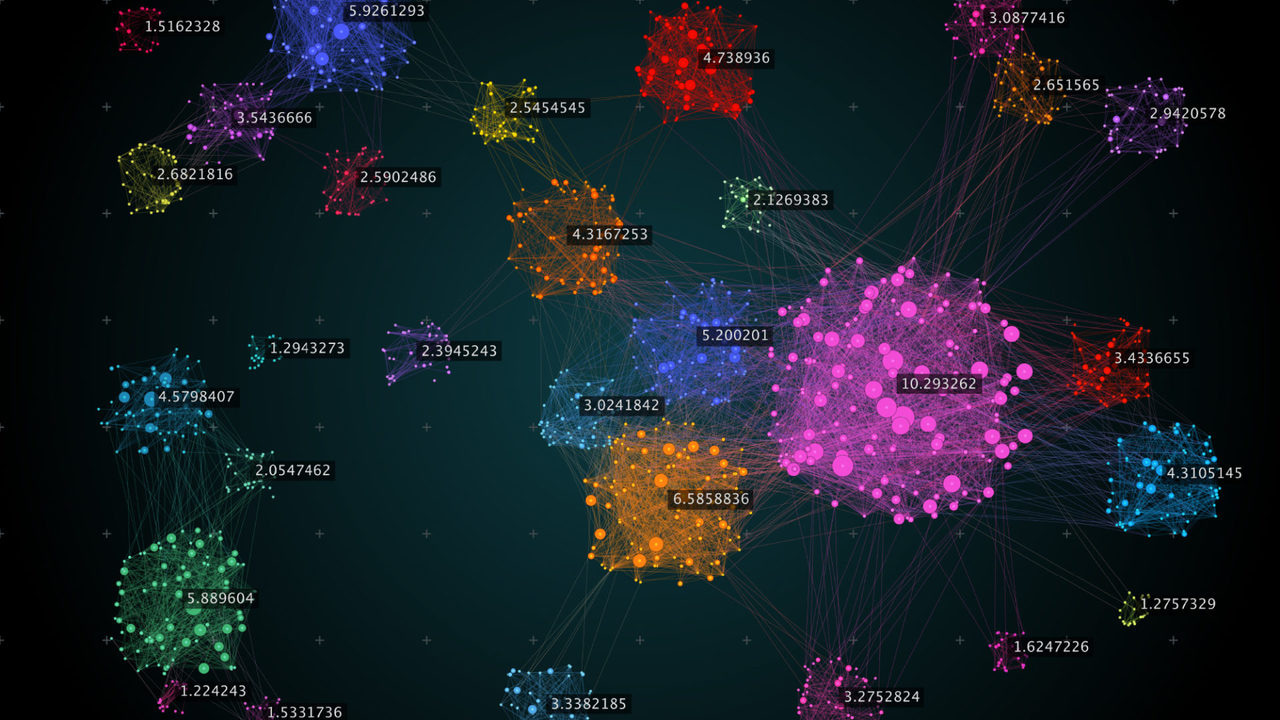Vegetation data analysis is one of the crucial issues we deal with in almost all researches we do in vegetation Science. One of the most common vegetation data analyses is the clustering analysis. In this article, I am going to focus on the clustering tendency issue.

When it comes to understanding and identifying the vegetation communities in a certain area, we often tend to use one, or two, of the different methods of clustering analyses. We would directly dive into using a certain software or code to do such a mission. However, have we ever considered the possibility that the data we are dealing with may not have meaningful clusters?! One of the issues of clustering algorithms and software is that they will manage to locate and specify clusters in data even if there are none (Cross & Jain 1982).

So, I think it is a crucial issue to measure the clustering tendency at the beginning of any clustering analysis; it will give an idea about the presence or absence of clusters in the data, and to which limit are the data clustered.

There are two common methods to assess the clustering tendency:
1. Hopkins’ statistical hypothesis test.
2. Visual Assessment of cluster Tendency (VAT).

In this article, I am going to focus mainly on the first method, Hopkins’ statistical hypothesis test that was proposed by Hopkins & Skellam (1954). The Hopkins’ test is used to assess the clustering tendency of a data set by measuring the probability that this data set is generated by a uniform data distribution. In other words, it tests the spatial randomness of the data.

For instance, let DS be a real data set of vegetation data plots or records. We can apply the Hopkins hypothesis test as next:

The Hopkins’ test formula can be written as next:

1. We take a number (n) of sample plots (p1 to pn) from the data set (DS).

1. For each sample plot (pi), we find the nearest neighbor one (pj) then calculate the distance (xi) between pi and pj. This can be annotated by this function:
xi = dist(pi,pj).

1. We make a simulated data set (sDS) containing number (n) of artificial plots (q1 to qn) drawn from a uniform distribution and with the same variation as the original real data set DS.

1. For each artificial plot (qi) in sDS we find its nearest neighbor real plot (qj) in DS, then we calculate the distance (yi) between qi and qj. This can be annotated by this function:
yi = dist(qi,qj).

1. We calculate the Hopkins statistic (H) as the mean nearest neighbor distance in the simulated data set sDS divided by the sum of the mean nearest neighbor distances in the real and simulated data sets.

The Hopkins’ test formula can be written as next: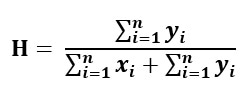So how to interpret this formula?
If the original data (DS) were uniformly distributed, then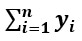and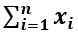would have close values, and thus H would be close to 0.5. On the other hand, if there are clusters present in DS, then the distances for artificial plotswould be much larger than those for the real onesand hence, the value of H will be larger than 0.5. If the value of H is larger than 0.75, this would indicate a clustering tendency at the 90% confidence level.

The null and the alternative hypotheses for Hopkins’ test are defined as follow:

• Null hypothesis (H0): the data set DS is uniformly distributed implying no meaningful clusters.
• Alternative hypothesis (H1): the data set DS is not uniformly distributed implying that it contains meaningful clusters.

To measure the clustering tendency of the data set of my research, I have developed a Python script to calculate the Hopkins’ statistical test value. The resulted value was 0.96 as shown in the Python code below. Since H value was larger than 0.5 and quite close to 1, I rejected, with more than 90% level of confidence, the null hypothesis and confirmed that the data set had clusters.

The  python code to calculate Hopkins' Test value:

The first 4 lines of the code below are to import the data set into Python compiler and wrangle it: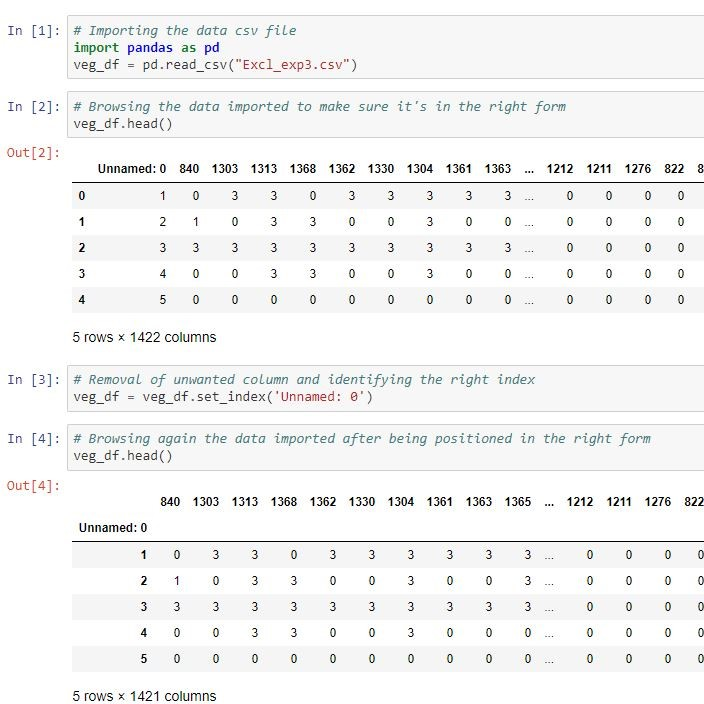The code section below is to build a function that calculates the Hopkins' Test value: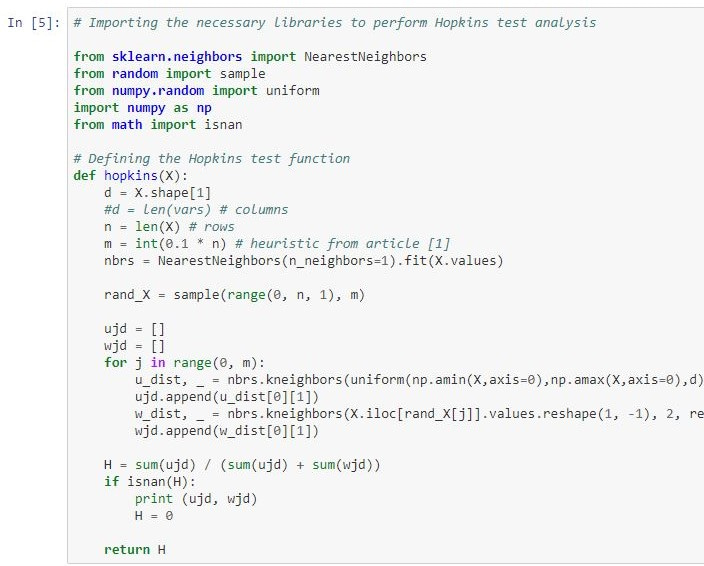The final code line below is to apply the built Hopkins' Test function into the imported data set: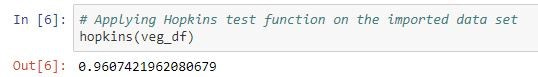As shown above, the hypothesis test value is 0.96 which is quite close to 1, so we can reject the null hypothesis and this means that the data set is non-uniformly distributed implying the presence of clusters.

I have made the code available for everyone and You can get it from the GitHub repository below:

https://github.com/mhatim99/Hopkins-Test

References:

Cross, G. R., & Jain, A. K. (1982). Measurement of clustering tendency. In Theory and Application of Digital Control (pp. 315-320). Pergamon.

Hopkins, B., & Skellam, J. G. (1954). A new method for determining the type of distribution of plant individuals. Annals of Botany, 18(2), 213-227.